# Monte-Carlo Glauber Model Simulations of Nuclear Collisions¶

Cal Poly San Luis Obispo

A senior project completed in collaboration with Dr. Jennifer Klay

June 13, 2014

## Abstract¶

In order to understand the geometry of nuclear collisions, an iPython-based simulation of the Monte-Carlo Glauber model was created. The simulation utilizes nuclear charge density distributions to create nuclei and cross-section data from the Particle Data Group to generate large samples of nuclear collisions. The simulation correlates the number of nucleons participating in a collision as well as the number of binary collisions as a function of the impact parameter for each event. Good agreement between the program and expected results for Au+Au collisions at beam energies $\sqrt{s_{NN}}$ = 200 GeV is demonstrated. The program also makes predictions on future RHIC experiments including He3+Au collisions at $\sqrt{s_{NN}}$ = 200 GeV. Individual collisions can be visually represented, demonstrating the event-by-event variation of particular geometric overlaps, which are obscured in the ensemble data.

## Introduction¶

The nucleus is composed of a bound state of protons and neutrons, collectively called nucleons, which are held together by the strong nuclear force. In the collision of nuclei of different elements, the interacting nucleons are scattered and transformed according to physical law, but the events occur so rapidly on scales so small that it is nigh impossible to preemptively determine how many nucleons will interact in the collision. The Glauber Model, developed by Roy Glauber, is a technique used to predict the number of binary collisions and participating particles as a function of impact parameter, the vector joining the centers of the two nuclei.

The two types of Glauber models include the Optical-limit approximation and Monte Carlo model. The optical approach makes several assumptions about the colliding nuclei that allow one to create analytical expressions to describe the impact and determine the number of interacting nucleons along with the number of binary collisions. The Monte Carlo Glauber model follows a more stochastic approach by distributing nucleons radially following a nuclear charge density profile unique to each element. The nucleons are given random azimuthal and polar angles. The separation distance of nucleons in the two overlapping nuclei is then compared to the inelastic cross-section to determine which individual nucleons participate in the collision. This approach assumes the nucleons travel straight throughout the entire event and can collide with many opposing nucleons without altering their paths. The simulations in this report exclusively use the Monte Carlo approach to Glauber modeling.

## Monte Carlo Approach¶

The Monte Carlo method is a geometric approach that requires the nuclear charge distributions in order to build realistic nuclei to collide. Several methods can be used to create this distribution (listed below) all of which are well defined thanks to De Vries’ et al. 1987 paper "Nuclear Charge Density by Elastic Electron Scattering". An example is the two parameter Fermi model that is used to create 197-Au nuclei, where a Woods-Saxon density profile is created from a mean field potential on the nucleons. The equation describes the force felt by each nucleon, and the potential can be utilized to map out a probability function for the radial position of each nucleon.

In all methods, a distribution function, $\rho(r)$, is sampled from to give each nucleon in the nucleus a certain radial position, $r$. The nucleon is then assigned random azimuthal and polar angles that allow the nucleus to be built in a three-dimensional, spherical coordinate system. In order to reduce processing time, the spherical coordinates of each nucleon are converted into cartesian coordinates and saved in the nucleus arrays. When sampling from the radial distribution, the distribute1D function samples $4\pi r^2 \rho(r)$ so that no radial position on the sphere is more likely to be chosen than another. The polar angle is obtained from transforming a uniform sample on the interval [-1,1] with the arccosine function and the azimuthal angle is sampled from a flat distribution over the range $–\pi$ to $\pi$. This gives an even distribution over the entire sphere that prevents oversampling near the poles. In essence, sampling in this fashion is the spherical equivalent to sampling over a uniform function in one dimension.

The interaction distance at which two nucleons can be considered to have collided is also needed to run the program. This distance is directly related to the inelastic cross section of the nucleons, itself a function of beam energy. Since we are only colliding ions, we need to understand the most basic ion collision: proton-proton. If one can accurately model a proton-proton collision, then one can model heavier ion collisions as a conglomeration of many individual proton or neutron collisions. The particle data group gathers large amounts of data about elastic and total proton-proton cross sections from many experiments, and compiles all this data in one compact source. This program pulls the data in real time and fits curves to both elastic and total cross section as a function of beam energy. The proton-proton inelastic cross section, $σ_{inel}^{pp}$, is given by the curve of the total cross section minus the elastic cross section. The cross section is converted to a radial distance using the equation that relates area of a circle to radius: $σ_{inel}^{pp}=\pi r^2$ or $r=\sqrt{σ_{inel}^{pp}/\pi}$.

This program correlates number of interacting particles ("participants") and binary nucleon-nucleon collisions to the impact parameter, $b$, which is simply the distance between the centers of the nuclei during the collision. Because we are assuming every individual nucleon travels straight throughout the collision, the impact parameter can be drawn as the distance between the centers of the nuclei when projected onto a flat plane.

The extracted participant and binary collision data can be used to gain insight on the geometry of nuclear collisions, such as those taking place at the Brookhaven Relativistic Heavy Ion Collider (RHIC) and the CERN Large Hadron Collider (LHC). A common application of the Glauber model is to correlate the number of produced particles ("multiplicity") observed by an experiment with the impact parameter of the collision by mapping percentiles of experimental multiplicity with percentiles of simulated participants/binary collisions. The monotonic relationship of number of participants/collisions with impact parameter is the fundamental assumption. Figure 1 shows the Monte Carlo Glauber results for RHIC collisions from Ref. , upon which this program is based. The relation between number of participating nucleons, $N_{part}$, number of collisions, $N_{coll}$, and impact parameter, $b$ for gold-gold (Au+Au) and copper-copper (Cu+Cu) collisions at center-of-mass energies, $\sqrt{S_{NN}}$, of 200 GeV is evident.## Program Details¶

The code is entirely open source, native to iPython, importable, and freely available on Github at https://github.com/MCGlauber/MCG. Emphasis was put on accumulating all the necessary data needed to run these types of simulations with ease of use. The following steps outline how to run the program for Au+Au and Cu+Cu collisions at 200 GeV to reproduce the results seen in Figure 1.

In :
%pylab inline

Populating the interactive namespace from numpy and matplotlib

In :
from GlauberModel import *
#Imports the main library file

2014 total cross section data is unavailable. The Particle Data Group website may not have the latest data or may have changed format.
Using 2013 data for total cross section.
Using 2013 data for elastic cross section.


The Particle Data Group does not regularly update the files with new data, so quite often we will be using data that may be up to a year old. Because there is already a vast storage of data, not having the most cutting edge information does not make a huge difference in the end results.

The DisplayData functions displays the most recent data downloaded from the Particle Data Group website as well as the curves fit to the total, elastic, and inelastic cross section. The inelastic cross section data is found by subtracting a best fit of the elastic cross section data from the total cross section data. Several points from TOTEM and ALICE at CERN that directly measure inelastic cross section are shown for comparison.[3,4,5,6]

In :
DisplayData()
#(Ref : Beringer et al. PDG)
#(Ref : Abelev et al. ALICE)
#(Ref [5,6]: Antchev et al. TOTEM)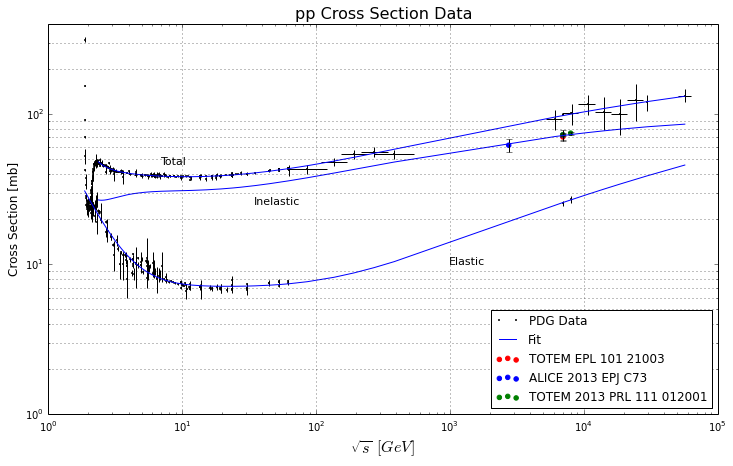The Collider function is the core of the program and requires several parameters to be defined.

N: The number of iterations will determine how many data points will be generated, but will also increase the run time. For 1,000 Au+Au collisions at 200 GeV, the program takes approximately 20 minutes to run.

Particle 1 and 2: The ions that will be collided.

A1 and A2: The number of nucleons in the colliding particles. Defining these explicitly helps the program separate different isotopes of the same element.

Energy: The beam energy in GeV which determines the inelastic cross section for the interaction. The program supports any beam energy between 3 and 60,000 GeV.

bRange: The range at which the impact parameter, $b$, can be drawn. A bRange of 1 means that the largest impact parameter possible will be equal the to sum of the radius of both nuclei, the most peripheral collision.

Model 1 and 2: The models used to determine the nuclear charge density distributions for the ions that will be undergoing collisions. From Ref . The models included are:

• HO : The harmonic-oscillator model.
• MHO: The modified harmonic-oscillator model.
• MI : Model-independent evaluation of the form factor.
• FB : Model-independed analysis by means of a Fourier-Bessel expansion.
• SOG: Model-independed analysis by means of an expansion for the charge distribution as a sum of Gaussians.
• 2pF: Two-parameter Fermi model.
• 3pF: Three-parameter Fermi model.
• 3pG: Three-parameter Gaussian model.
• UG : Uniform Gaussian model.

Range: The number of root-mean-square radii at which nucleons can be placed in a nucleus. A range of 2 guarantees the full radius of the nucleus. Larger values of the range will give more realistic nuclei but less precise nucleon placement.

Bins: The number of bins in which a nucleon can be placed into a nuclear radius. More bins give more realistic nuclei but takes longer to simulate.

In :
#The following parameters shows 1000 Au+Au collisions at 200 GeV
N=1000
Particle1='197Au'
Particle2='197Au'
A1=197
A2=197
Energy=200 #GeV
bRange=1.1
model1='2pF'
model2='2pF'
Range=2
Bins=100
help(Collider) #Shows the documentation for the Collider function

Help on function Collider in module GlauberModel:

Collider(N, Particle1, A1, Particle2, A2, model1, model2, Energy, bRange=1.1, Range=2, Bins=100)
Simulates N collisions between specified Elements (with number of nucleons A)
and using Center of Mass Energy [GeV].
Returns the matrices corresponding to center-to-center seperation distance
(Random value between 0 and bRange*(radius of nucleus1 + radius of nucleus2) [fm]),
Nuclei 1 and 2, number of participating nucleons, and the number of binary
collisions. Additionally returns the interaction distance of the nucleons
given the choosen beam energy and the radii of both nuclei.



When the Collider function is run, it returns the data that will be used to display the results.

In :
b,Nucleus1,Nucleus2,Npart,Ncoll,Maxr,Rp1,Rp2=Collider(N,Particle1,A1,Particle2,A2,model1,model2,Energy,bRange,Range,Bins)


Once the collider function has finished running, the arrays containing the data can be utilized using the PlotNuclei, ShowCollision, and PlotResults functions.

The PlotNuclei function graphs the radial density distribution of both particles. This is the distribution that the distribute1d function samples from.

In :
help(PlotNuclei)

Help on function PlotNuclei in module GlauberModel:

PlotNuclei(Nucleus1, Nucleus2, Particle1, Particle2, model1, model2, Rp1, Rp2, Range, Bins)
Plots the nuclear charge density for each nucleus and
shows the root-mean-square radius.
Blue corresponds to nucleus 1 and green to nucleus 2.


In :
PlotNuclei(Nucleus1,Nucleus2,Particle1,Particle2,model1,model2,Rp1,Rp2,Range,Bins)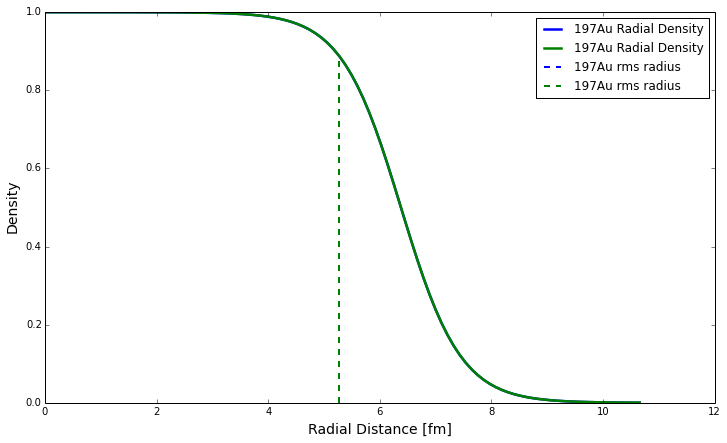The ShowCollision function plots the colliding nucleons with an outline of the rms radius of the nuclei.

In :
help(ShowCollision)

Help on function ShowCollision in module GlauberModel:

ShowCollision(N, Particle1, A1, Particle2, A2, Rp1, Rp2, Nucleus1, Nucleus2, b, Npart, Ncoll, Maxr)
Plots a cross-sectional and horizontal view of the last collision.


In :
ShowCollision(N,Particle1,A1,Particle2,A2,Rp1,Rp2,Nucleus1,Nucleus2,b,Npart,Ncoll,Maxr)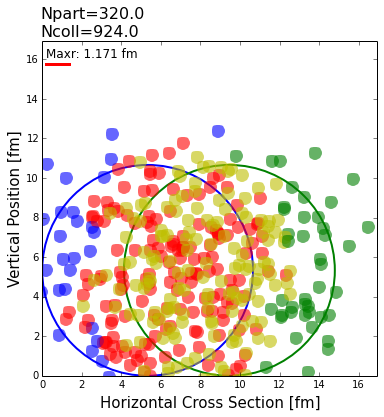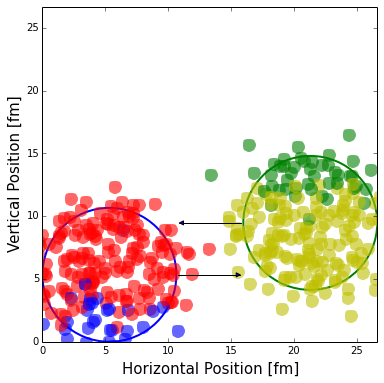The red and yellow points are nucleons that have collided with other nucleons. Blue and green nucleons escaped the collision unwounded.

The PlotResults function plots the number of binary collisions and participating particles versus the impact parameter as well as the average value for every femtometer.

In :
help(PlotResults)

Help on function PlotResults in module GlauberModel:

PlotResults(b, Npart, Ncoll, Particle1, Particle2, N, Energy, bins=10)
Plots number of collisions and participants as a function of impact parameter.
Shows average trend over data using specified number of bins.


In :
PlotResults(b,Npart,Ncoll,Particle1,Particle2,N,Energy,27)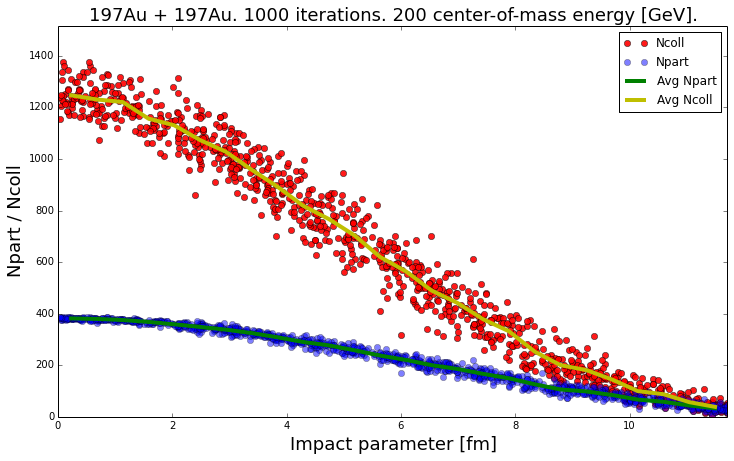These results match those from Ref.  for RHIC Au+Au collisions. As a double check the results for Cu+Cu collisions at 200 GeV are generated and shown below.

In :
N=1000;Particle1='63Cu';Particle2='63Cu';A1=63;A2=63;Energy=200;bRange=1.1;model1='2pF';model2='2pF';Range=2;Bins=100
b,Nucleus1,Nucleus2,Npart,Ncoll,Maxr,Rp1,Rp2=Collider(N,Particle1,A1,Particle2,A2,model1,model2,Energy,bRange,Range,Bins)
PlotResults(b,Npart,Ncoll,Particle1,Particle2,N,Energy,22)

Multiple parameters detected for specified model. Using primary values.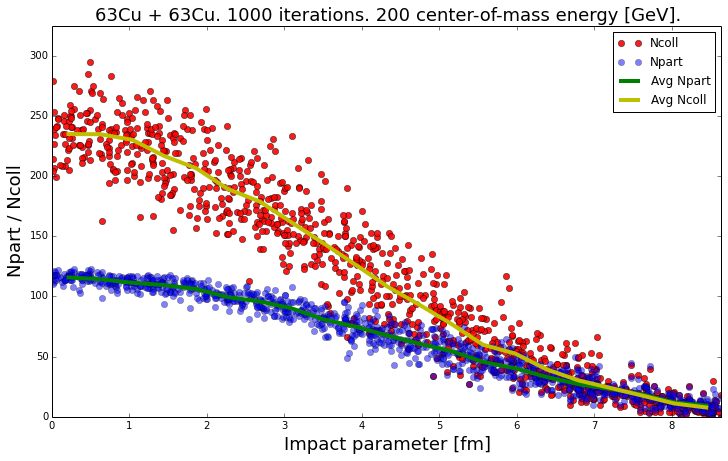Because the two-parameter fermi model for Cu-63 has two different sets of parameters, the program alerts us and defaults to the first set. However, once again, the results of Figure 1 are reproduced, allowing one to be confident in the accuracy of the program.

## Analysis¶

RHIC will also be performing He-3 and Au-197 collisions at 200 GeV in the future. The program can easily simulate these types of collisions to provide expected outcomes of the collisions.

In :
N=1000;Particle1='197Au';Particle2='3He';A1=197;A2=3;Energy=200;bRange=1.1;model1='2pF';model2='FB';Range=2;Bins=100
b,Nucleus1,Nucleus2,Npart,Ncoll,Maxr,Rp1,Rp2=Collider(N,Particle1,A1,Particle2,A2,model1,model2,Energy,bRange,Range,Bins)
PlotResults(b,Npart,Ncoll,Particle1,Particle2,N,Energy,40)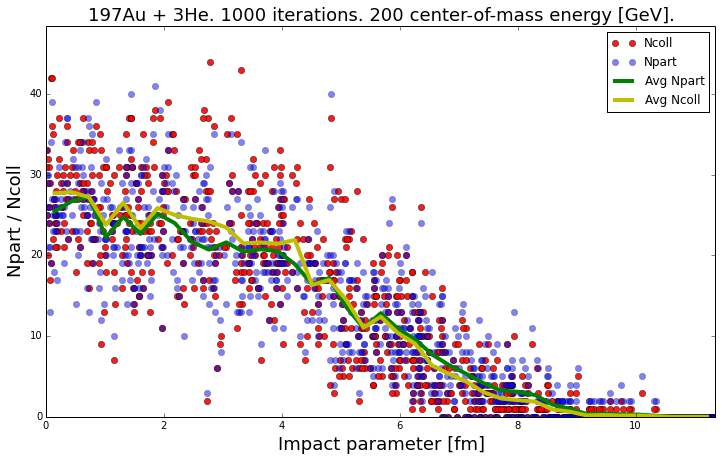For any collision, we expect more binary collisions to occur on average for smaller impact parameter. In this case we see a large spread in number of collisions and number of participants for similar impact parameter, a phenomenon that is regularly observed in the collision of ions of greatly different sizes. These inherent fluctuations signify that the orientation and geometry of the ions upon impact greatly influences the specific result of any given collision. For example, with impact parameter equal zero, the variation in $N_{part}$ and $N_{coll}$ is of order $\pm$10/27 or 37.0%. The average quantities (green and yellow lines) still show the expected trend as a function of impact parameter. The wide variation in $N_{part}$ and $N_{coll}$ for any specific collision is also illustrated in the example collisions shown below.

We contrast this to gold-gold collisions, where the variation is much narrower and the impact parameter is the primary determining factor for number of collisions observed. The specific geometry of a given nucleus has much less of an effect. The typical variation for zero impact parameter Au+Au collisions is $\pm$90/1260 or 7.14%. Nevertheless, there is interesting physics in these fluctuations and has been the subject of vigorous experimental investigation.

We also observe near perfect correspondence between average number of participants and collisions. This is a consequence of strongly asymmetric collisions where one of the colliding ions has only a few nucleons. In this case, because there are only three helium nucleons that are usually spread far from one another, when a gold nucleon does interact, it can usually only interact with one He3 nucleon so that one collision only adds one participant (the gold nucleon since the helium nucleus can add at most 3 participants). As a result, the number of participants and collisions stays roughly the same. As we collide heavier ions, nucleons in opposing ions can interact with many other nucleons throughout the collision, so one participant can see many collisions, leading to a much larger number of collisions than the number of participants at small impact parameters.

In :
ShowCollision(N,Particle1,A1,Particle2,A2,Rp1,Rp2,Nucleus1,Nucleus2,b,Npart,Ncoll,Maxr)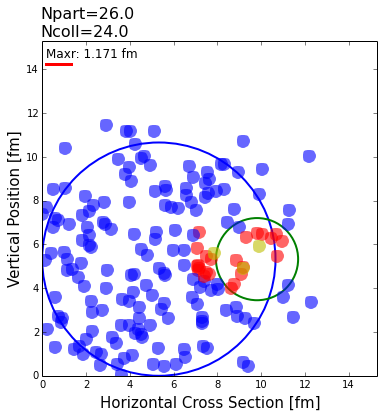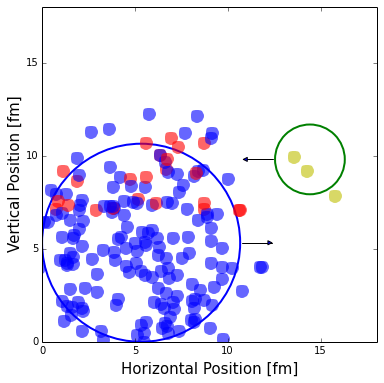The figure above shows a He-3 and Au-197 collision with an impact parameter of 5 fm. Nucleon geometry plays a large role in determining the number of collisions. A relatively close impact might not generate many collisions if only two of the three helium nucleons pass through the core of the gold nucleus. We could detect many more collisions from a peripheral impact as long as all three helium nucleons happened to pass through the core of the gold nucleus. This would require the three nucleons in the helium nucleus to be given large radial distances and similar azimuthal coordinates. The helium-3 radial density is shown below, illustrating that the reason why the average trend is decreasing is due to the fact that it is extremely unlikely that all three nucleons will be given similar angular positions and even less likely that they are sampled from large radial distances. We would expect only a few such events in a simulation of thousands of collisions.

In :
PlotNuclei(Nucleus1,Nucleus2,Particle1,Particle2,model1,model2,Rp1,Rp2,Range,Bins)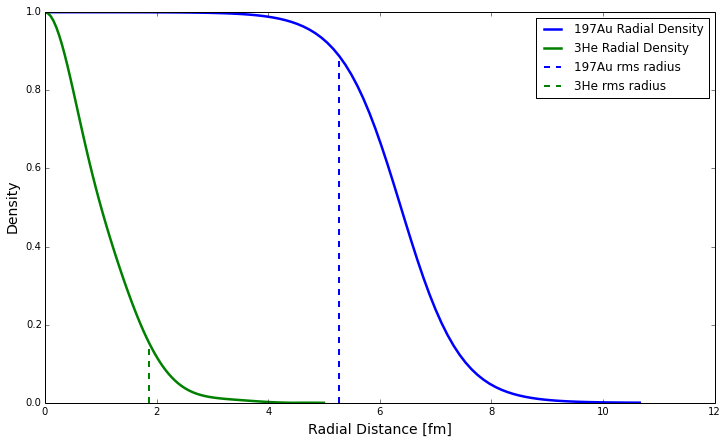The distribution for helium-3 is sampled from the absolute value of the Fourier-Bessel model for its nuclear charge density.[2,7] While it makes no physical sense for the density to be negative, the Fourier-Bessel model does go briefly negative within the maximum cut-off radius. However, the probability to be sampled from these regions is negligible. Similarly to all the other models, the distribution is sampled with a factor of $4\pi r^2$ and given random azimuthal and polar angles as described previously.

## Future Work¶

Currently the code contains support for a few different radial density models including the two and three parameter Fermi and Gaussian models, as well as partial support for the Fourier-Bessel model. In the future, we'd like to be able to have support for all models to build nuclei of the full list of ions available.

The program is also presently restricted to simulating nuclei according to a spherical shape. However, not all nuclei are spherical. For example, the Radon nucleus is pear shaped and Uranium is ellipsoidal. In order to provide support for these types of nuclei, the program would need to be modified to sample from non-uniform angular distributions and to keep track of the orientation of the entire nucleus upon collision. In addition, the definition of impact parameter would need to be modified since the non-spherical nucleus would have a center that varies with orientation.

In the future, we also plan to convert the code into a compiled library to optimize the processing time.

## Conclusions¶

The program provides an easy way for any user to accurately simulate collisions between many different types of heavy ions. Due to the sheer number of elements that can be simulated, there are possibly some collision combinations that have never been seen yet and interesting phenomena yet to be discovered. We hope that this program illuminates our understanding of nuclear collisions and proves to be useful in the future.

 Miller, M. L., Reygers, K., Sanders, S. J., Steinberg, P. Glauber Modeling in High Energy Nuclear Collisions, Ann. Rev. Nucl. Part. Sci. 57, 205-243 (2007). arXiv:nucl-ex/0701025

 De Vries, H., De Jager, C. W., De Vries, C., Nuclear Charge-Density-Distribution Parameters from Elastic Electron Scattering, Atom. Data Nucl. Data Tabl. 36:495 (1987). ScienceDirect

 J. Beringer et al. (Particle Data Group), Proton Elastic and Total Cross Section Data, Phys. Rev. D86, 010001 (2012). http://pdg.lbl.gov/2012/hadronic-xsections/

 B. Abelev, et al. (ALICE collaboration), Measurement of inelastic, single and double diffraction cross sections in proton-proton collisions at LHC with ALICE, Eur. Phys. J. C 73:2456 (2013). arXiv:hep-ex/1208.4968

 G. Antchev et al., (The TOTEM Collaboration), Measurement of proton-proton inelastic scattering cross-section at ${\sqrt {s} = 7\,{\mathrm {TeV}}}$, EPL 101 21003 (2013). doi:10.1209/0295-5075/101/21003

 G. Antchev et al., (The TOTEM Collaboration), Luminosity-Independent Measurement of the Proton-Proton Total Cross Section at ${\sqrt {s} = 8\,{\mathrm {TeV}}}$, Phys. Rev. Lett. 111, 012001 (2013). APS

 Martensson-Pendrill, A.-M., Gustavsson, M. G. H. The Atomic Nucleus, from the Handbook of Molecular Physics and Quantum Chemistry, (ISBN 0 471 62374 1), John Wiley and Sons, Ltd, Chichester (2003) http://physics.gu.se/~f3aamp/tl.pdf

# Appendix¶

Code can be inspected using "load magic"

In :
%load GlauberModel.py

In [ ]:
import IPython.core.pylabtools as pyt
import numpy as np
import matplotlib.pyplot as plt
from datetime import date
from urllib2 import urlopen
from scipy.optimize import curve_fit
import time

#Importing data from particle data group
#Attempts to use data from current year
#If that data is not available, drops down a year until data is found or defaults to 2013 data
Y=date.today().year
i=0
while i<=Y-2013:
try:
print "Using "+str(Y-i)+" data for total cross section."
DataFound1=True
break
except:
print str(Y-i)+" total cross section data is unavailable. The Particle Data Group website may not have the latest data or may have changed format."
i+=1
if i>Y-2013:
DataFound1=False
l=0
while l<=Y-2013:
try:
print "Using "+str(Y-l)+" data for elastic cross section."
DataFound2=True
break
except:
l+=1
if l>Y-2013:
DataFound2=False

if DataFound1==True:
Point=data[:,0]
Plab=data[:,1] #GeV/c
Plab_min=data[:,2]
Plab_max=data[:,3]
Sig=data[:,4]
StEr_H=data[:,5]
StEr_L=data[:,6]
SyEr_H=data[:,7]
SyEr_L=data[:,8]
if DataFound2==True:
EPoint=Edata[:,0]
EPlab=Edata[:,1] #GeV/c
EPlab_min=Edata[:,2]
EPlab_max=Edata[:,3]
ESig=Edata[:,4]
EStEr_H=Edata[:,5]
EStEr_L=Edata[:,6]
ESyEr_H=Edata[:,7]
ESyEr_L=Edata[:,8]

pyt.figsize(12,7)

def Ecm(Plab):
"""Converts Plab momenta to center of mass energy [GeV]."""
E=(((Plab**2+.938**2)**(1/2.)+.938)**2-(Plab**2))**(1/2.)
return E
if DataFound1==True and DataFound2==True:
#Automatically converts all P_lab momenta to corresponding center-of-mass energy [GeV]
E_cm=Ecm(Plab)
eE_cm=Ecm(EPlab)
cm_min=Ecm(Plab_min)
cm_max=Ecm(Plab_max)
ecm_min=Ecm(EPlab_min)
ecm_max=Ecm(EPlab_max)

#Define best fit curve given by the particle data group
def func(s,P,H,M,R1,R2,n1,n2):
m=.93827 #Proton mass GeV/c^2
sM=(2*m+M)**2 #Mass^2 (GeV/c^2)^2
hbar=6.58211928*10**-25 #GeV*s
c=2.99792458*10**8 #m/s
sigma=H*(np.log(s**2/sM))**2+P+R1*(s**2/sM)**(-n1)-R2*(s**2/sM)**(-n2)
return sigma

#Apply best fit curve to the elastic cross-section data
s=eE_cm[:]
y=ESig[:]
p0=[4.45,.0965,2.127,11,4,.55,.55]
popt,pcov=curve_fit(func,s,y,p0)

#Apply best fit curve to total cross-section data
s2=E_cm[90:]
y2=Sig[90:]
p0=[34.49,.2704,2.127,12.98,7.38,.451,.549]
popt2,pcov2=curve_fit(func,s2,y2,p0)

def SigI(BE):
"""Returns the proton-proton cross-sectional area [fm^2] for given beam energy [GeV]"""
return .1*(func(BE,popt2,popt2,popt2,popt2,popt2,popt2,popt2)-func(BE,popt,popt,popt,popt,popt,popt,popt))

def DisplayData():
"""Displays the Proton-Proton Cross Section Data."""
plt.loglog(E_cm,Sig,ls=' ',marker='.',markersize=3,color='black',label='PDG Data')
plt.loglog(eE_cm,ESig,ls=' ',marker='.',markersize=3,color='black')
plt.loglog(E_cm[90:],func(E_cm[90:],popt2,popt2,popt2,popt2,popt2,popt2,popt2),color='blue')
plt.loglog(E_cm,func(E_cm,popt,popt,popt,popt,popt,popt,popt),color='blue',label='Fit')
plt.scatter(7000,70.5,label='TOTEM EPL 101 21003',color='red')
plt.scatter([2760,7000],[62.1,72.7],label='ALICE 2013 EPJ C73',color='blue')
plt.scatter([7000,8000],[72.9,74.7],label='TOTEM 2013 PRL 111 012001 ',color='green')
plt.errorbar([2760,7000,7000,7000,8000],[62.1,70.5,72.7,72.9,74.7],yerr=[5.9,3.4,6.2,1.5,1.7],fmt=' ',color='black')
plt.loglog(E_cm[90:],10*SigI(E_cm[90:]))
plt.errorbar(E_cm,Sig,xerr=[E_cm-cm_min,cm_max-E_cm],yerr=[StEr_L,StEr_H],ms=.5,mew=0,fmt=None,ecolor='black')
plt.errorbar(eE_cm,ESig,xerr=[eE_cm-ecm_min,ecm_max-eE_cm],yerr=[EStEr_L,EStEr_H],ms=.5,mew=0,fmt=None,ecolor='black')
plt.annotate("Total",fontsize=11,xy=(7,46),xytext=(7,46))
plt.annotate("Elastic",fontsize=11,xy=(1000,10),xytext=(1000,10))
plt.annotate("Inelastic",fontsize=11,xy=(35,25),xytext=(35,25))
plt.title("pp Cross Section Data",fontsize=16)
plt.ylabel("Cross Section [mb]",fontsize=12)
plt.xlabel("$\sqrt{s}\,[GeV]$",fontsize=16)
plt.ylim(1,400)
plt.grid(which='minor',axis='y')
plt.grid(which='major',axis='x')
plt.legend(loc=4)
plt.show()

#Reads in parameters to calculate nuclear charge densities (NCD)
pNucleus=parameters[:,0]
pModel=parameters[:,1]
pr2=parameters[:,2]
pC_A=parameters[:,3]
pZ_Alpha=parameters[:,4]
pw=parameters[:,5]
FBnucleus=FBdata[:,0]
FBrms=FBdata[:,1]
FBR=FBdata[:,2]
FBa=np.zeros((len(FBnucleus)-1,17),float)
for i in range(len(FBnucleus)-1):
FBa[i,:]=FBdata[i+1,3:]
FBa = FBa.astype(np.float)

def NCD(Nucleus,Model,Range=2,Bins=100):
"""Returns the Nuclear Charge Distribution for a given Nucleus with specified
model. Creates radial distribution from 0 to Range*nuclear radius with n
number of bins. If no values are set, defaults to 197Au using two-parameter
Fermi model up to twice the nuclear radius with 100 bins."""
#For multiple models of the same nucleus takes the first set of parameters and notifies the user which parameters are used.
j=[]
for index in range(len(pNucleus)):
if pNucleus[index]==Nucleus and pModel[index]==Model:
j.append(index)
i=index
j=np.array(j,dtype=int)
if len(j)>1:
#print "Multiple parameters detected for given model. Using primary values."
i=j
r=np.linspace(0,Range*float(pr2[i]),Bins)
if Model=='HO':
return (1+float(pZ_Alpha[i])*(r/float(pC_A[i]))**2)*np.exp(-1*(r/float(pC_A[i]))**2)
elif Model=='MHO':
print "Warning: Model not yet supported\nPlease use a different model."
return None
elif Model=='Mi':
print "Warning: Model not yet supported\nPlease use a different model."
return None
elif Model=='FB':
#print "Warning: Fourier-Bessel Model currently contains support for H-3, He-3, C-12, and O-16 only. If not these ions, please choose another model."
for FBindex in range(len(FBnucleus)):
if FBnucleus[FBindex]==Nucleus:
iFB=FBindex
r=np.linspace(0,float(FBR[iFB]),Bins)
p=np.zeros(np.size(r),float)
v=np.arange(0,17,1)
for i in range(len(r)):
p[i]=abs(sum(FBa[iFB-1,v]*np.sinc((v+1)*r[i]/float(FBR[iFB]))))
return p
elif Model=='SOG':
print "Warning: Model not yet supported\nPlease use a different model."
return None
elif Model=='2pF':
return 1/(1+np.exp((r-float(pC_A[i]))/float(pZ_Alpha[i])))
elif Model=='3pF':
return (1+float(pw[i])*r**2/float(pC_A[i])**2)/(1+np.exp((r-float(pC_A[i]))/float(pZ_Alpha[i])))
elif Model=='3pG':
return (1+float(pw[i])*r**2/float(pC_A[i])**2)/(1+np.exp((r**2-float(pC_A[i])**2)/float(pZ_Alpha[i])**2))
elif Model=='UG':
print "Warning: Model not yet supported\nPlease use a different model."
return None
else:
print 'Error: Model not found\nPlease check that the model was typed in correctly. (Case Sensitive)'
return None

def distribute1D(x,prob,N):
"""Takes any numerical distribution probability, on
the interval defined by the array x, and returns an
array of N sampled values that are statistically the
same as the input data."""
y=prob*4*np.pi*x**2
A=np.cumsum(y)/(np.cumsum(y)[(len(x)-1)])
z=np.random.random_sample(N)
B=np.searchsorted(A,z)
return x[B]

def Collider(N,Particle1,A1,Particle2,A2,model1,model2,Energy,bRange=1.1,Range=2,Bins=100):
"""
Simulates N collisions between specified Elements (with number of nucleons A)
and using Center of Mass Energy [GeV].
Returns the matrices corresponding to center-to-center seperation distance
(Random value between 0 and bRange*(radius of nucleus1 + radius of nucleus2) [fm]),
Nuclei 1 and 2, number of participating nucleons, and the number of binary
collisions. Additionally returns the interaction distance of the nucleons
given the choosen beam energy and the radii of both nuclei.
"""
#Set Rp1 and Rp2 equal to the radii of the nuclei choosen
j1=[]
j2=[]
i1="Unassigned"
i2="Unassigned"
for index in range(len(pNucleus)):
if pNucleus[index]==Particle1 and pModel[index]==model1:
j1.append(index)
i1=index
if pNucleus[index]==Particle2 and pModel[index]==model2:
j2.append(index)
i2=index
j1=np.array(j1,dtype=int)
j2=np.array(j2,dtype=int)
if len(j1)>1 or len(j2)>1:
print "Multiple parameters detected for specified model. Using primary values."
i1=j1
i2=j2
if i1=="Unassigned" or i2=="Unassigned":
print 'Error: Model not found\nPlease check that the model was typed in correctly. (Case Sensitive)'
return None,None,None,None,None,None,None,None
if model1 != 'FB':
Rp1=float(pr2[i1])
else:
for FBindex in range(len(FBnucleus)):
if FBnucleus[FBindex]==Particle1:
iFB=FBindex
Rp1=float(FBR[iFB])
if model2 != 'FB':
Rp2=float(pr2[i2])
else:
for FBindex in range(len(FBnucleus)):
if FBnucleus[FBindex]==Particle2:
iFB=FBindex
Rp2=float(FBR[iFB])
b=(Rp1+Rp2)*bRange*np.random.random_sample(N) #Create array of random impact parameters
if model1 != 'FB':
r1=np.linspace(0,Range*Rp1,Bins) #Array of radial data used for plotting
else:
r1=np.linspace(0,Rp1,Bins)
if model2 != 'FB':
r2=np.linspace(0,Range*Rp2,Bins)
else:
r2=np.linspace(0,Rp2,Bins)
Npart=np.zeros(N,float)
Ncoll=np.zeros(N,float)
Maxr=np.sqrt(SigI(Energy)/np.pi) #Radius within which two nucleons will interact
#Runs N number of times; each run creates a new array containing the parameters of interest: Ncoll, Npart, etc.
for L in range(N):
Nucleus1=np.zeros((A1,7),float)
Nucleus2=np.zeros((A2,7),float)
#Gives each nucleon is own radial distance from the center of the nucleus
#Sampled from the NCD function and distributed with a factor 4*pi*r^2*p(r)
Nucleus1[:,0]=distribute1D(r1,NCD(Particle1,model1,Range,Bins),A1)[:]
Nucleus2[:,0]=distribute1D(r2,NCD(Particle2,model2,Range,Bins),A2)[:]
#Nucleons are then given random azimuthal distances such that no particular point is more likely to be populated than another
#Cartesian coordinates (x,y,z) are determined from spherical coordinates and passed to the nuclei arrays
for i in range(A1):
Nucleus1[i,1]=np.arccos(2*np.random.random_sample(1)-1)
Nucleus1[i,2]=2*np.pi*np.random.random_sample(1)
Nucleus1[i,3]=Nucleus1[i,0]*np.sin(Nucleus1[i,1])*np.cos(Nucleus1[i,2])
Nucleus1[i,4]=Nucleus1[i,0]*np.sin(Nucleus1[i,1])*np.sin(Nucleus1[i,2])
Nucleus1[i,5]=Nucleus1[i,0]*np.cos(Nucleus1[i,1])
for i in range(A2):
Nucleus2[i,1]=np.arccos(2*np.random.random_sample(1)-1)
Nucleus2[i,2]=2*np.pi*np.random.random_sample(1)
Nucleus2[i,3]=Nucleus2[i,0]*np.sin(Nucleus2[i,1])*np.cos(Nucleus2[i,2])
Nucleus2[i,4]=Nucleus2[i,0]*np.sin(Nucleus2[i,1])*np.sin(Nucleus2[i,2])
Nucleus2[i,5]=Nucleus2[i,0]*np.cos(Nucleus2[i,1])
for p1 in range(A1):
for p1x in range(A1):
FailSafe=0 #Prevents program from running indefinitely in some cases
if p1x==p1:
pass
else:
while np.sqrt((Nucleus1[p1,3]-Nucleus1[p1x,3])**2+(Nucleus1[p1,4]+Nucleus1[p1x,4])**2+(Nucleus1[p1,5]+Nucleus1[p1x,5])**2)<Maxr:
Nucleus1[p1x,1]=np.arccos(2*np.random.random_sample(1)-1)
Nucleus1[p1x,2]=2*np.pi*np.random.random_sample(1)
Nucleus1[p1x,3]=Nucleus1[p1x,0]*np.sin(Nucleus1[p1x,1])*np.cos(Nucleus1[p1x,2])
Nucleus1[p1x,4]=Nucleus1[p1x,0]*np.sin(Nucleus1[p1x,1])*np.sin(Nucleus1[p1x,2])
Nucleus1[p1x,5]=Nucleus1[p1x,0]*np.cos(Nucleus1[p1x,1])
FailSafe+=1
if FailSafe>10:
Nucleus1[p1x,0]=distribute1D(r1,NCD(Particle1,model1,Range,Bins),A1)[p1x]
for p2 in range(A2):
for p2x in range(A2):
FailSafe=0
if p2x==p2:
pass
else:
while np.sqrt((Nucleus2[p2,3]-Nucleus2[p2x,3])**2+(Nucleus2[p2,4]-Nucleus2[p2x,4])**2+(Nucleus2[p2,5]-Nucleus2[p2x,5])**2)<Maxr:
Nucleus2[p2x,1]=np.arccos(2*np.random.random_sample(1)-1)
Nucleus2[p2x,2]=2*np.pi*np.random.random_sample(1)
Nucleus2[p2x,3]=Nucleus2[p2x,0]*np.sin(Nucleus2[p2x,1])*np.cos(Nucleus2[p2x,2])
Nucleus2[p2x,4]=Nucleus2[p2x,0]*np.sin(Nucleus2[p2x,1])*np.sin(Nucleus2[p2x,2])
Nucleus2[p2x,5]=Nucleus2[p2x,0]*np.cos(Nucleus2[p2x,1])
FailSafe+=1
if FailSafe>10:
Nucleus2[p2x,0]=distribute1D(r2,NCD(Particle2,model2,Range,Bins),A2)[p2x]
for p1 in range(A1): #Loops through all nucleons in Nucleus 1
count=0 #Arbitrary count variable used to determine whether a nucleon escaped the collision unwounded
for p2 in range(A2): #Loops through all nucleons in Nucleus 2
if np.sqrt((Nucleus1[p1,3]-Nucleus2[p2,3])**2+(b[L]+Nucleus2[p2,4]-Nucleus1[p1,4])**2) <= Maxr:
Ncoll[L]+=1
#If distance between nucleons is smaller than maxr, nucleons interact and 1 collision is counted.
if np.sqrt((Nucleus1[p1,3]-Nucleus2[p2,3])**2+(b[L]+Nucleus2[p2,4]-Nucleus1[p1,4])**2) > Maxr:
count+=1
#If distance is greater than maxr, +1 is added to count
if count==A2:
#If count reaches the number of total nucleons in nucleus 2, we can infer that
#the nucleon in nucleus 1 has not hit any other nucleons in the other nucleus. Therefore
#this nucleon is flagged as an unwounded particle
Nucleus1[p1,6]=1
for p2 in range(A2):
count=0
for p1 in range(A1):
if np.sqrt((Nucleus1[p1,3]-Nucleus2[p2,3])**2+(b[L]+Nucleus2[p2,4]-Nucleus1[p1,4])**2) > Maxr:
count+=1
if count==A1:
Nucleus2[p2,6]=1
#Number of participating particles is the total number of particles minus all the flagged unwounded particles
Npart[L]=A1+A2-(sum(Nucleus1[:,6])+sum(Nucleus2[:,6]))
if model1 == 'FB':
Rp1=float(pr2[i1])
if model2 == 'FB':
Rp2=float(pr2[i2])
return b,Nucleus1,Nucleus2,Npart,Ncoll,Maxr,Rp1,Rp2

def PlotNuclei(Nucleus1,Nucleus2,Particle1,Particle2,model1,model2,Rp1,Rp2,Range,Bins):
"""
Plots the nuclear charge density for each nucleus and
shows the root-mean-square radius.
Blue corresponds to nucleus 1 and green to nucleus 2.
"""
r1=np.linspace(0,Range*Rp1,Bins)
r2=np.linspace(0,Range*Rp2,Bins)
if model1 != 'FB':
Range1=Range
else:
for FBindex in range(len(FBnucleus)):
if FBnucleus[FBindex]==Particle1:
iFB=FBindex
RpFB1=float(FBR[iFB])
Range1=1
r1=np.linspace(0,RpFB1,Bins)
if model2 != 'FB':
Range2=Range
else:
for FBindex in range(len(FBnucleus)):
if FBnucleus[FBindex]==Particle2:
iFB=FBindex
RpFB2=float(FBR[iFB])
Range2=1
r2=np.linspace(0,RpFB2,Bins)
d1=NCD(Particle1,model1,Range,Bins)/max(NCD(Particle1,model1,Range,Bins))
d2=NCD(Particle2,model2,Range,Bins)/max(NCD(Particle2,model2,Range,Bins))
rms1=r1[np.abs(r1-Rp1).argmin()]
rms2=r2[np.abs(r2-Rp2).argmin()]
plt.ylabel("Density",fontsize=14)
plt.legend()
plt.show()

def ShowCollision(N,Particle1,A1,Particle2,A2,Rp1,Rp2,Nucleus1,Nucleus2,b,Npart,Ncoll,Maxr):
"""Plots a cross-sectional and horizontal view of the last collision."""
fig,ax=plt.subplots()
N1=plt.Circle((Rp1,Rp1),Rp1,color='b',fill=False,lw=2)
N2=plt.Circle((Rp1+b[N-1],Rp1),Rp2,color='g',fill=False,lw=2)
for i in range(A1):
if Nucleus1[i,6]==1:
ax.plot(Rp1+Nucleus1[i,4],Rp1+Nucleus1[i,3],'b.',ms=26,alpha=.6,mew=0,mec='blue')
if Nucleus1[i,6]==0:
ax.plot(Rp1+Nucleus1[i,4],Rp1+Nucleus1[i,3],'r.',ms=26,alpha=.6,mew=0,mec='red')
for i in range(A2):
if Nucleus2[i,6]==1:
ax.plot(b[N-1]+Rp1+Nucleus2[i,4],Rp1+Nucleus2[i,3],'g.',ms=26,alpha=.6,mew=0,mec='green')
if Nucleus2[i,6]==0:
ax.plot(b[N-1]+Rp1+Nucleus2[i,4],Rp1+Nucleus2[i,3],'y.',ms=26,alpha=.6,mew=0,mec='yellow')
zed=1.5*(Rp1+Rp2)+b[N-1]
ax.annotate('Npart='+str(Npart[N-1])+'\nNcoll='+str(Ncoll[N-1]),xy=(1,0),xytext=(0,1.015*zed),fontsize=16)
ax.annotate('Maxr: '+str(Maxr)[:5]+' fm',xy=(0,2*Rp1),xytext=(.01*zed,.95*zed),fontsize=12)
ax.plot([(.01*zed),(.01*zed)+Maxr],[zed*.93,zed*.93],color='r',ls='-',lw=3)
plt.xlim((0,zed))
plt.ylim((0,zed))
plt.xlabel('Horizontal Cross Section [fm]',fontsize=15)
plt.ylabel('Vertical Position [fm]',fontsize=15)
fig.set_size_inches(6,6)
fig1,ax1=plt.subplots()
N3=plt.Circle((Rp1,Rp1),Rp1,color='b',fill=False,lw=2)
N4=plt.Circle(((Rp1+Rp2)*2,Rp1+b[N-1]),Rp2,color='g',fill=False,lw=2)
for i in range(A1):
if Nucleus1[i,6]==1:
ax1.plot(Rp1+Nucleus1[i,5],Rp1+Nucleus1[i,4],'b.',ms=26,alpha=.6,mew=0,mec='blue')
if Nucleus1[i,6]==0:
ax1.plot(Rp1+Nucleus1[i,5],Rp1+Nucleus1[i,4],'r.',ms=26,alpha=.6,mew=0,mec='red')
for i in range(A2):
if Nucleus2[i,6]==1:
ax1.plot(2*(Rp1+Rp2)+Nucleus2[i,5],b[N-1]+Rp1+Nucleus2[i,4],'g.',ms=26,alpha=.6,mew=0,mec='green')
if Nucleus2[i,6]==0:
ax1.plot(2*(Rp1+Rp2)+Nucleus2[i,5],b[N-1]+Rp1+Nucleus2[i,4],'y.',ms=26,alpha=.6,mew=0,mec='yellow')
ax1.annotate("",xy=(2*Rp1+Rp2,Rp1), xycoords='data',xytext=(2*Rp1,Rp1), textcoords='data',arrowprops=dict(arrowstyle='-|>',connectionstyle="arc"))
ax1.annotate("",xy=(2*Rp1,b[N-1]+Rp1), xycoords='data',xytext=(2*(Rp1+Rp2)-Rp2, b[N-1]+Rp1), textcoords='data',arrowprops=dict(arrowstyle='-|>',connectionstyle="arc"))
zed=2.5*(Rp1+Rp2)
plt.xlim((0,zed))
plt.ylim((0,zed))
plt.ylabel('Vertical Position [fm]',fontsize=15)
plt.xlabel('Horizontal Position [fm]',fontsize=15)
fig1.set_size_inches(6,6)
plt.show()

def PlotResults(b,Npart,Ncoll,Particle1,Particle2,N,Energy,bins=10):
"""Plots number of collisions and participants as a function of impact parameter.
Shows average trend over data using specified number of bins."""
xmin=0
xmax=max(b)
x=b
y=Ncoll
j = bins
E = np.zeros(j)
H = np.zeros(j)
L = np.zeros(j)#,int64)
newx = np.linspace(xmin,xmax,j)
binwidth = (xmax-xmin)/float(j)
#Shift by half a bin so the values plot at the right location?
#If the bins are small enough or the function not too steep, this doesn't matter
plotx = newx - 0.5*binwidth
for i in range(len(x)):
#find the array element in newx where the value from x belongs
val = x[i]
bin = newx.searchsorted(val)
L[bin] += 1
E[bin] += y[i]**2
H[bin] += y[i]
h = H/L
spr = np.sqrt(E/L - h**2)
err = spr/np.sqrt(L)
y2=Npart
E2 = np.zeros(j)
H2 = np.zeros(j)
L2 = np.zeros(j)#,int64)
newx2 = np.linspace(xmin,xmax,j)
binwidth2 = (xmax-xmin)/float(j)
plotx2 = newx2 - 0.5*binwidth2
for i in range(len(x)):
val2 = x[i]
bin = newx2.searchsorted(val2)
L2[bin] += 1
E2[bin] += y2[i]**2
H2[bin] += y2[i]
h2 = H2/L2
spr2 = np.sqrt(E2/L2 - h2**2)
err2 = spr2/np.sqrt(L2)
plt.plot(x,y,"ro",alpha=.9,label='Ncoll')
plt.plot(x,y2,"bo",alpha=.5,label='Npart')
plt.plot(plotx2,h2,"g-",linewidth=4,label='Avg Npart')
plt.plot(plotx,h,"y-",linewidth=4,label='Avg Ncoll')
plt.xlim(0,max(x))
plt.ylim(0,1.1*max(y))
plt.legend()
plt.ylabel('Npart / Ncoll', fontsize=18)
plt.xlabel('Impact parameter [fm]', fontsize=18)
plt.title(str(Particle1)+' + '+str(Particle2)+'. '+str(N)+' iterations. '+str(Energy)+' center-of-mass energy [GeV].', fontsize=18)
plt.show()